# The TTEST Procedure

#### Common Notation

Table 119.5 displays notation for some of the commonly used symbols.

Table 119.5: Common Notation

Symbol

Description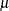Population value of (arithmetic) mean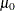Null value of test (value of H0= option in PROC TTEST statement)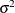Population variance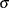Population value of standard deviation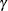Population value of geometric mean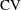Population value of coefficient of variation (ratio of population standard deviation and population arithmetic mean)
Value of ALPHA= option in PROC TTEST statement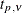pth percentile of t distribution with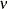degrees of freedom (df)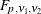pth percentile of F distribution with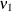numerator df and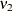denominator df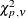pth percentile of chi-square distribution withdf